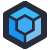John works for a manufacturing company which has accrued a large portfolio of C++ code. Developed over the course of decades, by many people, there’s more than a little legacy cruft and coding horrors mixed in. Frustrated with the ongoing maintenance, and in the interests of “modernization”, John was tasked with converting the legacy C++ into C#.

In the section for creating TPS reports, there were two functions, `TpsRound` and `TpsRound2`. The code between the two of them was nearly identical- someone had clearly copy/pasted and made minor tweaks.

``````CString EDITFORM::TpsRound2(double dbIntoConvert)
{
// This Stub calculates the rounding based
// upon this company standards as
// outlined in TPS 101 Conversion Rules and
// dual dimensioning practices

CString csHold1,csHold2;
int  decimal, sign,ChkDigit;
long OutVal;
char *buffer2;
char *outbuff;
outbuff = "                          ";
buffer2 = "                     ";

if (dbIntoConvert == 0)
{
//		return CString("0.00");
}

buffer2 = _fcvt( dbIntoConvert, 7, &decimal, &sign );
buffer2[decimal] = '.';

csHold2 = XvertDecValues(dbIntoConvert);

if (m_round	== FALSE)
{
return csHold2;
}

ChkDigit = atoi(csHold2.Mid(2,1));
OutVal = atol(csHold2.Left(2));

if (ChkDigit >= 5)
{
OutVal++;
}

if (OutVal >= 100)
{
buffer2[decimal] = '0';
decimal++;
buffer2[decimal] = '.';
}

ltoa(OutVal,outbuff,10);
buffer2=outbuff;
buffer2=outbuff;
int jj=2;  // this value is the ONLY difference to `TpsRound()`
while (jj < decimal)
{
buffer2[jj++]='0';
}
csHold1 = CString(buffer2).Left(decimal);
return csHold1;
}``````

At its core, this is just string-mangling its way through some basic rounding operations. Writing your own C++ rounding is less of a WTF than it might seem, simply because C++ didn’t include standard rounding methods for most of its history. The expectation was that you’d implement it yourself, for your specific cases, as there’s no one “right” way to round.

This, however, is obviously the wrong way. The code is actually pretty simple, just cluttered with a mix of terrible variable names, loads of string conversion calls, and a thick layer of not understanding what they’re doing.

To add to the confusion, `buffer2` holds the results of `_fcvt`- a method which converts a floating point to a string. `csHold2` holds the results of `XvertDecValues`, which also returns a floating point converted to a string, just… a little differently.

``````CString EDITFORM::XvertDecValues(double incon1)
{
char *buffer3;
char *outbuff;
int  decimal, sign;
buffer3 = "                     ";
outbuff = "00000000000000000000";
buffer3 = _fcvt(incon1, 7, &decimal, &sign );

if (incon1 == 0)
{
//		return CString("0.00");
}

int cnt1,cnt2,cnt3;
cnt1 = 0;
cnt2 = 0;

cnt3 = decimal;
if (cnt3 <= 0)
{
outbuff[cnt2++]='.';
while (cnt3 < 0)
{
outbuff[cnt2++]='0';
cnt3++;
}
}
else
{

while (cnt1 < decimal)
{
outbuff[cnt2] = buffer3[cnt1];
cnt1++;
cnt2++;
}
outbuff[cnt2] = '.';
cnt2++;

}

while (cnt1 < 15)
{
outbuff[cnt2] = buffer3[cnt1];
cnt1++;
cnt2++;
}

outbuff[cnt2] = '\0';

return CString(outbuff);
}``````

So, back in `TpsRound2`, `csHold2` and `buffer2` both hold a string version of a floating point number, but they both do it differently. They’re both referenced. Note also the check `if (OutVal >= 100)`- `OutVal` holds the leftmost two characters of `csHold2`- so it will never be greater than or equal to 100.

John’s orders were to do a 1-to–1 port of functionality. “Get it working in C#, then we can refactor.” So John did. And then once it was working in C#, he threw all this code away and replaced it with calls to C#’- built in rounding and string formatting methods, which were perfectly fine for their actual problems.[Advertisement] ProGet’s got you covered with security and access controls on your NuGet feeds. Learn more.